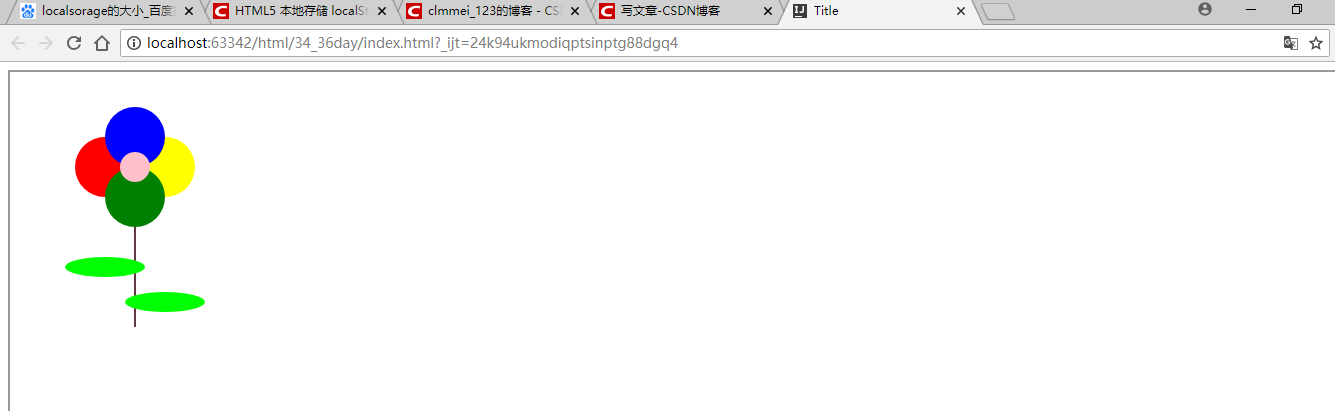SVG画图功能：svg实现画出一朵花（附代码）

<canvas> 标记和 SVG 以及 VML 之间的一个重要的不同是，<canvas> 有一个基于 JavaScript 的绘图 API，而 SVG 和 VML 使用一个 XML 文档来描述绘图。

<?xml version="1.0" standalone="no"?>

<!DOCTYPE svg PUBLIC "-//W3C//DTD SVG 1.1//EN"
"http://www.w3.org/Graphics/SVG/1.1/DTD/svg11.dtd">

<svg width="100%" height="100%" version="1.1"
xmlns="http://www.w3.org/2000/svg">

<circle cx="100" cy="50" r="40" stroke="black"
stroke-width="2" fill="red"/>

</svg>

SVG使用XML编写，并保存为.svg文件。该.svg文件必须在.html文件中被引用

1）使用<embed>标签：被所有主流的浏览器支持，并允许使用脚本

<embed src="rect.svg" width="300" height="100"
type="image/svg+xml"

pluginspage: 指向下载插件的url

2）使用object标签：html4的标准标签，被所有较新的浏览器支持，不允许使用脚本

<object data="rect.svg" width="300" height="100"
type="image/svg+xml"

codebase：指向下载插件的url

3)iframe标签：（推荐）

<iframe src="rect.svg" width="300" height="100">
</iframe>

1、矩形<rect width="" height="" style="">

<rect width="300" height="100"
style="fill:rgb(0,0,255);stroke-width:1;
stroke:rgb(0,0,0)"/>

style 属性用来定义 CSS 属性

stroke-width 属性定义矩形边框的宽度

stroke 属性定义矩形边框的颜色

2、圆形<circle>

3、椭圆<ellipse>

<ellipse cx="300" cy="150" rx="200" ry="80"
style="fill:rgb(200,100,50);
stroke:rgb(0,0,100);stroke-width:2"/>

cx 属性定义圆点的 x 坐标

cy 属性定义圆点的 y 坐标

rx 属性定义水平半径（通过两个焦点的线的一半）

ry 属性定义垂直半径

4、线条<line>

5、多边形<polygon>

6、折线<polyline>

7、路径<path>

flower.svg

<?xml version="1.0" standalone="no"?>
<!DOCTYPE svg PUBLIC "-//W3C//DTD SVG 1.1//EN"
"http://www.w3.org/Graphics/SVG/1.1/DTD/svg11.dtd">

<svg width="100%" height="100%" version="1.1"
xmlns="http://www.w3.org/2000/svg">

<circle cx="90" cy="90" r="30" fill="red" />

<circle cx="150" cy="90" r="30" fill="yellow" />

<circle cx="120" cy="60" r="30" fill="blue" />

<circle cx="120" cy="120" r="30" fill="green" />
<circle cx="120" cy="90" r="15" fill="pink"/>

<line x1="120" y1="150" x2="120" y2="250"
style="stroke:rgb(100,55,69);stroke-width:2"/>

<ellipse cx="90" cy="190" rx="40" ry="10"
style="fill:lime"/>
<ellipse cx="150" cy="225" rx="40" ry="10"
style="fill:lime"/>
</svg>

index.html

<!DOCTYPE html>
<html lang="en">
<meta charset="UTF-8">
<title>Title</title>
<body>
<iframe src="flower.svg" width="100%" height="600" style="padding: 5px">
</iframe>
</body>
</html>HTML标签：img标签的用法总结

svg中<marker>元素的使用及marker属性的介绍

svg如何实现坐标系统变换（附代码）